Equable Spherical Cone

EQUABLE SPHERICAL CONE

Balmoral Software

Solutions: 1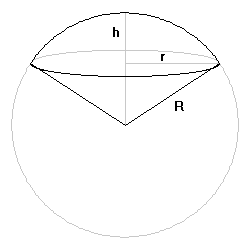A spherical cone consists of a spherical cap surmounting an inverted cone of the same lateral extent whose apex is at the center of the associated sphere. There is exactly one equable spherical cone.

Define the following notation:

h: Height of the spherical cap, 0 < h < R
r: Radius of the widest part, 0 < r < R
The volume of the spherical cone is V, where
V/π = (2/3)R2h
The lateral surface area of the upper portion is Scap, where
Scap/π = 2Rh
The lateral surface area of the lower portion is Scone, where
Scone/π = Rr
The above three quantities are integers by convention. Then
V/π = (R/3)(2Rh) = (R/3)(Scap/π),
so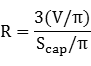is rational. We have
r2 = R2 - (R - h)2 = 2Rh - h2
The equability requirement is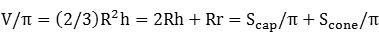2h(R - 3) = 3r

The spherical cone cannot be equable if R ≤ 3, so assume R > 3 and square both sides:
4h2(R - 3)2 = 9r2 = 9(2Rh - h2)

h[4(R - 3)2 + 9] = 18R

The coefficient of h is strictly positive and we can thus solve for h: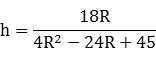Since R is rational, so is h. We then have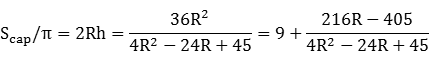The fractional part at right has a positive numerator since R > 3. Its denominator was previously established to also be positive, so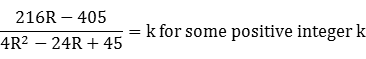4kR2 - 24(k + 9)R + 45(k + 9) = 0 

A solution for R requires that the discriminant of this quadratic be non-negative:
 Discriminant = 576(k + 9)2 - 720k(k + 9) ≥ 0 

1 ≤ k ≤ 36

We can then evaluate  to see which of the 36 possible values of k produces a square discriminant, as required by a rational solution for R in . There is only one such value, and the corresponding roots for R are checked for R > 3, and in  to see if a valid value of h in the range 0 < h < R is produced:
kDiscriminantQuadratic in R Roots for Rh
272162108R2 - 864R + 1620 = 03 (discard), 518/5
The single solution is a sphere with radius 5 and cap height 18/5, for which the volume and surface area are equal to 60 π:
R = 5
h = 18/5
r = 24/5
Scap = 36 π
Scone = 24 π
V = 60 π